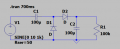# Greinacher circuit output voltage

#### Kandi123

Joined Apr 8, 2021
4Hey,
The task is to vary the load resistance (100k, 21k, 1.8k) and say why the output voltage is lower if i use a lower resistance.

In the next task i have to do the same without the resistance of the voltage source. In this case, it doesnt matter what load resistance i choose, the output voltage stays the same.

Now i have to explain that... But i have no clue tbh... Hope you can help me <3

#### Audioguru again

Joined Oct 21, 2019
4,933
The 50 ohms resistance of the voltage source obviously forms a voltage divider with the load resistance.
Your schematic writes it but does not show the source resistance.

#### Papabravo

Joined Feb 24, 2006
18,978
Do you know that using the default diode model is pretty far from reality.
Do you know what an RC time constant is and why it might be important here?
Do you know how to compute the reactance of a capacitor at a given frequency?
do you know how to step the value of R1?

Last edited:
•Kandi123

#### Kandi123

Joined Apr 8, 2021
4
Ok, after reading your answers i have a theory (That might be wrong)
For my question there are two answers.
The 50 ohms resistance of the voltage source obviously forms a voltage divider with the load resistance.
Thats the first one. If i lower the load resistance, theres a higher voltage drop on the 50 ohms resistance (it exists even if it doesnt show) and obviously a lower one on the load resistance. Without the 50 ohms resistance the output voltage doesnt change because theres only one part of the voltage divider left.

Do you know what an RC time constant is and why it might be important here?
Do you know how to compute the reactance of a capacitor at a given frequency?
do you know how to step the value of R1?
Thats the second reason (And yes i know about all these things, and it isnt for reality it is a theoretical task... I know about all these things in reality but i still cant use them in context if you know what i mean) is that with a lower Resistance, the discharge is faster. That means that the capacitor cant be completely loaded -> voltage drops

Is my theory correct?

#### Papabravo

Joined Feb 24, 2006
18,978
Ok, after reading your answers i have a theory (That might be wrong)
For my question there are two answers.

Thats the first one. If i lower the load resistance, theres a higher voltage drop on the 50 ohms resistance (it exists even if it doesnt show) and obviously a lower one on the load resistance. Without the 50 ohms resistance the output voltage doesnt change because theres only one part of the voltage divider left.

Thats the second reason (And yes i know about all these things, and it isnt for reality it is a theoretical task... I know about all these things in reality but i still cant use them in context if you know what i mean) is that with a lower Resistance, the discharge is faster. That means that the capacitor cant be completely loaded -> voltage drops

Is my theory correct?
Yes that is the first part of it. Now, by eliminating the series resistance you make the voltage source an "ideal" voltage source What is the major property of an ideal voltage source? How much current can it supply?

•Kandi123

#### Kandi123

Joined Apr 8, 2021
4
How much current can it supply?
As the voltage is a defined value and resistance 0... The current can be infinite in theory i think... But what exactly has that to do with my output voltage?

#### Papabravo

Joined Feb 24, 2006
18,978
As the voltage is a defined value and resistance 0... The current can be infinite in theory i think... But what exactly has that to do with my output voltage?
Well, the reason a real supply, with some internal resistance, cannot keep the capacitor charged is because it cannot supply enough current to keep it charged during the positive half cycle, with a heavy load resistor. the ideal supply can do that.

•Kandi123
Thx for your help i think i understand it now